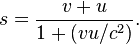# Relative speed equation

I am studying the relative speed equations but I confused in one point.
We need (at least) how many observers to calculate relativistic speed equation.
Three or two.

mfb
Mentor
What do you mean with "relativistic speed equation"?

You do not need observers, you can do this with pen and paper without observing actual experiments at all. It can be useful to imagine what multiple different observers would see, but they are not a necessary part for calculations.

I mean lets think theres two observers and nothing else.Can we use relativistic speed equation in these situations.

Relativistic speed equationDale
Mentor
2021 Award
To use that formula you need two reference frames (with relative velocity u) and one object (with velocity v relative to one frame).

Whether or not any of the frames are attached to an observer is not relevant. But if they are attached to an observer then the observer can certInly be used as the object.

Last edited: